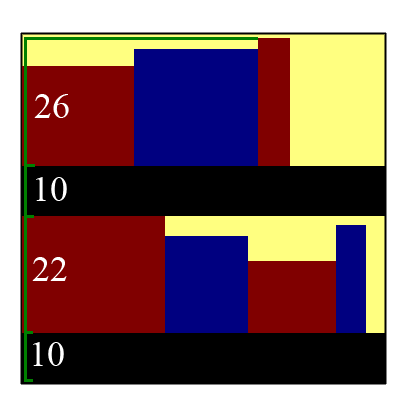Problem Statement

You have a single bookshelf in your home, and too many books! There is no way they will all fit, and some of them are going to have to go in the garage. Given the total collection of books, and the value of each, try to maximize the overall value of the books you can fit in your bookshelf.

The bookshelf has height H and width W. You can put in as many shelves in it as you want, but each shelf has height 10 (including the shelf on the bottom). You must place the books standing up, and the total width of the books on each shelf may not exceed W. Similarly, the total height must not exceed H. For example, if you place a book with height 22 on shelf 0, and a book with height 26 on shelf 1, the total height will be 10+22+10+26=68 (see image below).You will be given inputs bookHeights, bookWidths and bookValues, where corresponding elements give the height, width and value of each book. You should return a int[] containing an element for each book. If you elect to place a book in the garage, the entry for that book should be -1. Otherwise, indicate the index of the shelf you want to place the book on (starting from 0). Note that once you have determined which shelves to place the books on, the minimum height of each shelf is determined, and the validity of your solution can be determined automatically.

If you return an invalid arrangement, your score for that test case will be 0. An arrangement can be invalid if you don't return the right number of elements, if the shelf indices are less than -1 or greater than or equal to the number of books, or if the arrangement would result in a height greater than H or width greater than W. Your score for each test case with a valid return will simply be the value you manage to squeeze into the bookshelf, and your overall score will simply be the sum of your individual scores.

We've provided a visualization/offline testing tool here.

Definition

 Class: BookSelection Method: arrange Parameters: int, int, int[], int[], int[] Returns: int[] Method signature: int[] arrange(int H, int W, int[] bookHeights, int[] bookWidths, int[] bookValues) (be sure your method is public)

Notes

-You can imagine that the widths are all given in hundredths of an inch, while the heights are given in tenthes of inches.
-The time limit is 2 seconds. The memory limit is 1024M. There is no code size limit.

Constraints

-The height of each book will be randomly selected from a normal distribution with mean 80 and standard deviation A, where A is constant for each test case with a real value in [10,40]. After selection, the value will be rounded to the nearest integer.
-The width of each book will be selected similarly, except from a distribution with mean 200 and standard deviation in [50,200].
-Any width or height less than 1 in the above selection will be increased to 1.
-The value of each book will max(1,round(h*w*x)), where h and w are the width and height of the book, and x is a real value selected from a normal distribution with mean and standard deviation 0.001.
-W will be an integer selected uniformly at random in [2400,6000].
-H will be an integer selected uniformly at random in [240,1200].
-The number of books will be selected uniformly at random in [X,3*X], where X = H * W / 16000.

Examples

0)

 "1"
Returns: "H = 427<br>W = 5880<br>books = 212<br>seed = 1"
1)

 "2"
Returns: "H = 1075<br>W = 2507<br>books = 206<br>seed = 2"
2)

 "3"
Returns: "H = 1082<br>W = 4360<br>books = 703<br>seed = 3"
3)

 "4"
Returns: "H = 263<br>W = 4898<br>books = 213<br>seed = 4"
4)

 "5"
Returns: "H = 458<br>W = 4423<br>books = 147<br>seed = 5"
5)

 "6"
Returns: "H = 1011<br>W = 2422<br>books = 169<br>seed = 6"
6)

 "7"
Returns: "H = 397<br>W = 4180<br>books = 163<br>seed = 7"
7)

 "8"
Returns: "H = 474<br>W = 4052<br>books = 211<br>seed = 8"
8)

 "9"
Returns: "H = 333<br>W = 3086<br>books = 106<br>seed = 9"
9)

 "10"
Returns: "H = 870<br>W = 3974<br>books = 245<br>seed = 10"

Problem url:

http://www.topcoder.com/stat?c=problem_statement&pm=10885

Problem stats url:

http://www.topcoder.com/tc?module=ProblemDetail&rd=14227&pm=10885

Unknown

Search